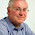## Friday, May 17, 2013

### Internet Explorer version ≤8 parseInt() octal radix default

Old browsers, like IE7 and IE8 ( that uses ECMAScript older than 5) uses octal radix ( or base ) as default when parsing strings that start with “0” as ints. Internet Explorer 8 and older versions therefore have this rather peculiar default behavior.

parseInt(“01”) => 1*8^0 = 1
parseInt(“05”) => 5*8^0 = 5
parseInt("055") => (5*8^1)+(5*8^0), 40+5 = 45
parseInt(“07”) => 7*8^0 = 7

parseInt(“08”) => 0*8^0 = 0 (the 8 has no equivalent in base 8)
parseInt(“09”) => 0, same issue

parseInt(“10”) => (0*10^0) + (1*10^1) = 10 ( IE8 recognizes this as base 10, as it doesn’t start with zero.)

Say that you're parsing months as ints, 01-12. 01-09 will be parsed as base 8, and 10-12 as base 10.
It'll work in all new browsers, but 08 and 09 will be parsed as zero in IE8 or older ( or other old browsers ).

Luckily, for us all, there is a way to specify which base to use.

parseInt(string,base);
parseInt(“08”,10) => 8*10^0 = 8

1.2.